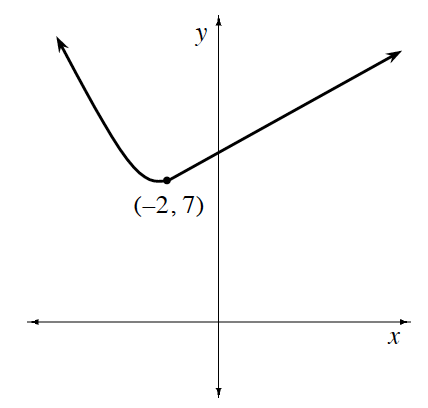### Home > APCALC > Chapter 2 > Lesson 2.1.2 > Problem2-24

2-24.

Given the graph of $y = h(x)$ at right, sketch:

1. $y = -h(x)$

vertical flip over $x$-axis

2. $y = h(x) - 5$

vertical shift, $5$ units down

3. $y = h^{-1}(x)$

inverse function, $x$- and $x$-values switch. Start with the known point.